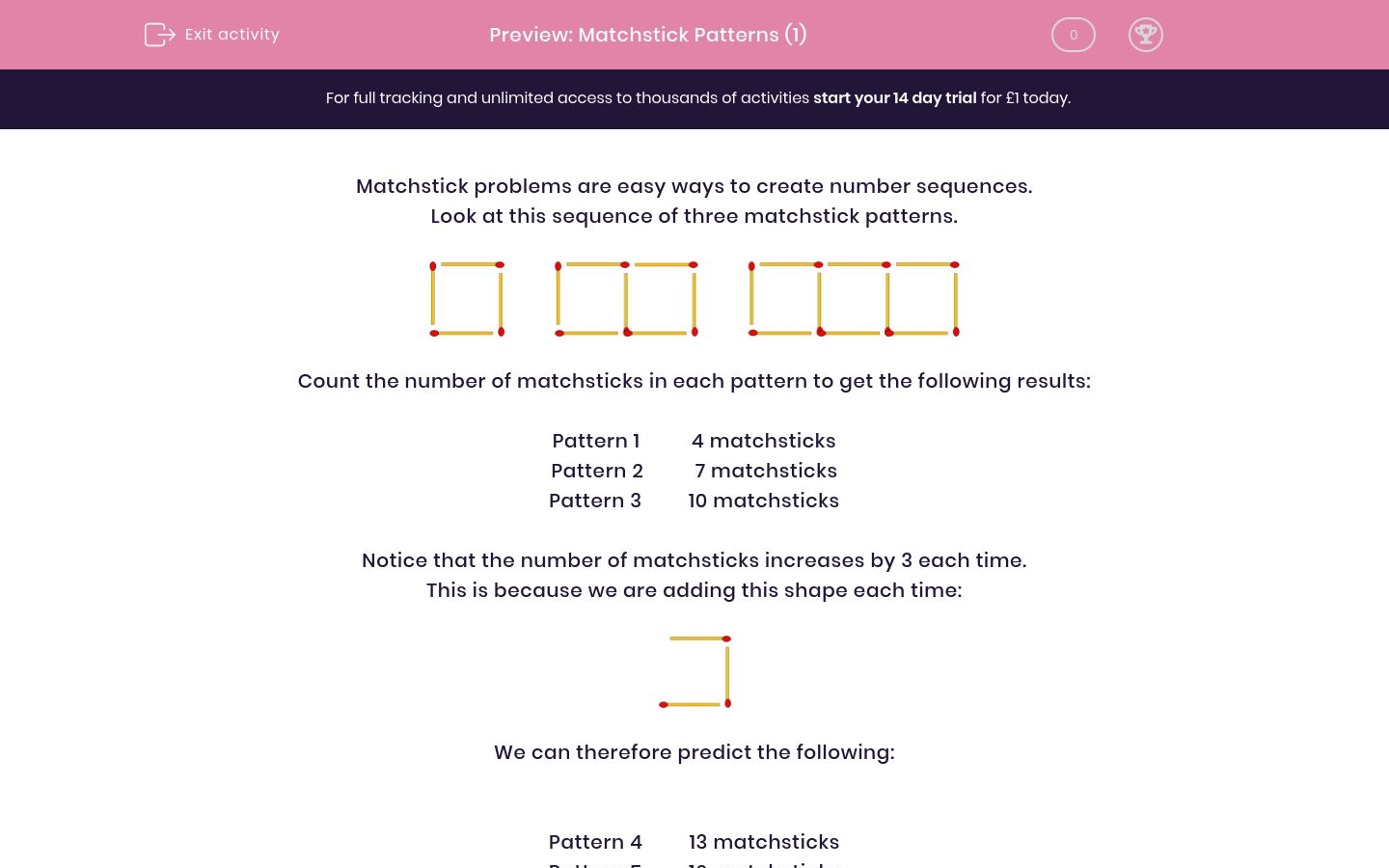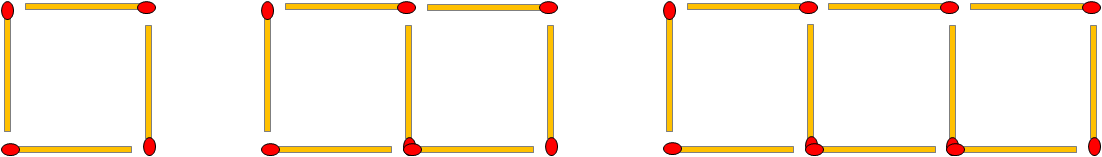# Matchstick Patterns (1)

In this worksheet, students link matchstick problems to number sequences.Key stage:  KS 2

Curriculum topic:   Maths and Numerical Reasoning

Curriculum subtopic:   Place Value

Difficulty level:### QUESTION 1 of 10

Matchstick problems are easy ways to create number sequences.

Look at this sequence of three matchstick patterns.Count the number of matchsticks in each pattern to get the following results:

Pattern 1          4 matchsticks

Pattern 2          7 matchsticks

Pattern 3         10 matchsticks

Notice that the number of matchsticks increases by 3 each time.

This is because we are adding this shape each time:We can therefore predict the following:

Pattern 4         13 matchsticks

Pattern 5         16 matchsticks

Pattern 6         19 matchsticks

---- OR ----